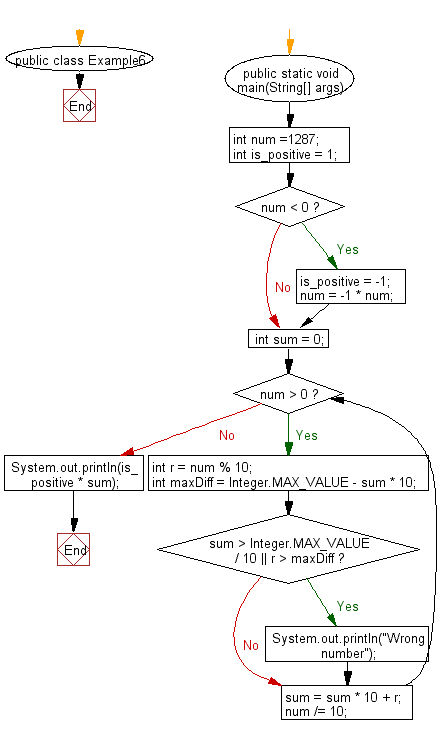﻿ Java Math Exercises: Reverse an integer number - w3resource# Java Math Exercises: Reverse an integer number

## Java Math Exercises: Exercise-6 with Solution

Write a Java program to reverse an integer number.

Sample Solution:

Java Code:

``````public class Example6 {
public static void main(String[] args) {
int num =1287;
int is_positive = 1;
if (num < 0) {
is_positive = -1;
num = -1 * num;
}
int sum  = 0;
while (num > 0) {
int r = num % 10;

int maxDiff = Integer.MAX_VALUE - sum * 10;
if (sum > Integer.MAX_VALUE / 10 || r > maxDiff)
System.out.println("Wrong number");;

sum = sum * 10 + r;
num /= 10;
}
System.out.println(is_positive * sum);
}
}
``````

Sample Output:

```7821
```

Flowchart:Java Code Editor:

What is the difficulty level of this exercise?

﻿

## Java: Tips of the Day

Parsing dates:

```import java.io.*;
import java.util.*;
import java.text.*;

String s = "2001/09/23 14:39";

SimpleDateFormat formatter = new SimpleDateFormat ("yyyy/MM/dd H:mm");
Date d = formatter.parse(s, new ParsePosition(0));
```